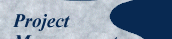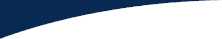Published here December 2004.

Brenda Taylor from South Africa Originally Wrote

Hi all

I had a very interesting discussion with a "thinker" yesterday and what he showed me, embarrassed me because I had never questioned something that never made sense to me anyway.

When calculating Earned Value (EV) of a project, we multiply the planned value (PV) by the percentage work complete (% Com). I know this because many many many many textbooks tell me this and so do many many many guides to the project management body of knowledge.

To calculate the schedule variance (SV) one subtracts the planned value from the earned value.

So EV = PV x % Com
SV = EV - PV

So SV = (PV x % Com.) - PV [derived by substituting PV x % Com for EV]

If a project has a budget of R10,000 and to date we had planned to spend R7,000 and we have spent R7,000 and the project is planned to be 70% complete and is 70% complete then the schedule variance and the cost variance should be zero because, although it is only 70% complete, we are in fact on schedule and there is therefore no variance evident in the schedule.

According to this formula, however, this is not true. If I were to input the values -- as indicated by the formula -- the schedule variance would reflect as follows:

EV=7,000 x 70%
= R4,900

So the SV = R4,900 - R7,000
= R2,100 See what I mean?

Okay, so let's work out the SPI, because we know that if the project is running according to schedule and budget, then the SPI should be 1 i.e. 100%, right?

SPI = EV/PV
=4900/7000

Which is 1 i.e. 100%.

So, the only real way of attaining the "correct" answer would be to use the % work complete of the planned % work complete. So, it would be more appropriate to use 100% complete in the above example because the project is, in fact, 100% on track and that is how you derive the value earned to the project by investing the R7,000 in the work performed. If this is the case, I would recommend that another step be added into the process to determine the % complete of the planned % complete i.e.:

Actual % work complete/Planned % work complete [expressed as a percentage] and then using this in the formula for calculating the EV.

Am I misunderstanding every single text book I have read or is there a minor flaw in the way the guru's have put the lessons into writing?

Any help would be most appreciated.

Kindest regards
Brenda Taylor
Senior Project Manager